About Qweas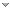RSS Feeds     BBS Forum Make Qweas.com My Home Page     Bookmark this page Register     Login     Help     Send Feedback
 All software Windows Mac Linux Mobile Games Screensavers Source Code Webware
 Related Search Terms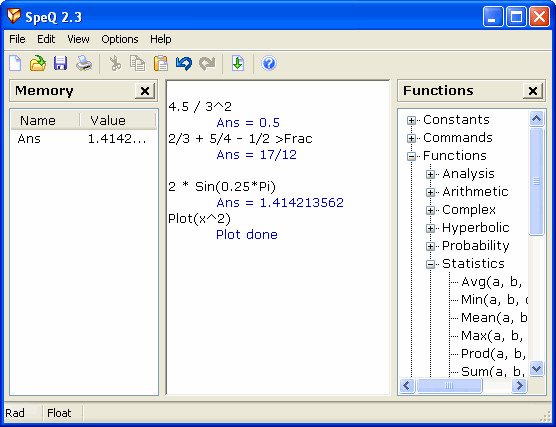## SpeQ Mathematics

SpeQ is a small, extensive mathematics program with a simple, intuitive interface. It can handle all kinds of scientific calculations. You can define variables and functions, and plot graphs of your functions. All calculations are entered in a sheet.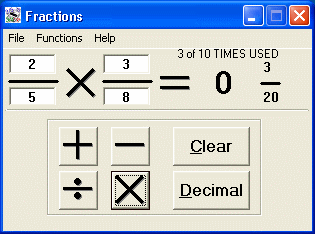## FRACTIONS N DECIMALS

FRACTIONS N DECIMALS is a helpful mathematics tool that is great for school and work. It is very easy to use. There are two programs in one. One application can be used to do Add, Subtract, Divide and Multiply fractions, and another can be used to ...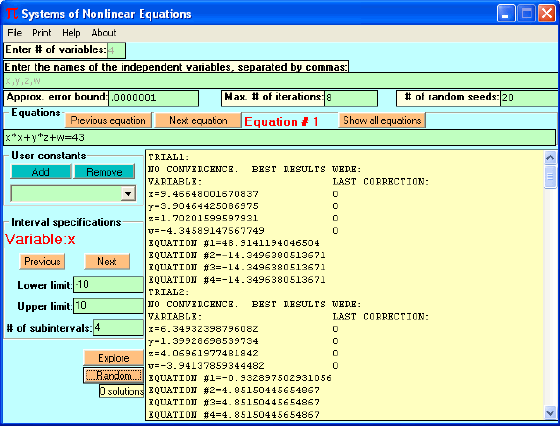## Systems of Nonlinear Equations

Systems of Nonlinear Equations numerically solves systems of simultaneous nonlinear equations. It can fully explore defined intervals to search for multiple solutions or quickly find solutions starting with random seeds.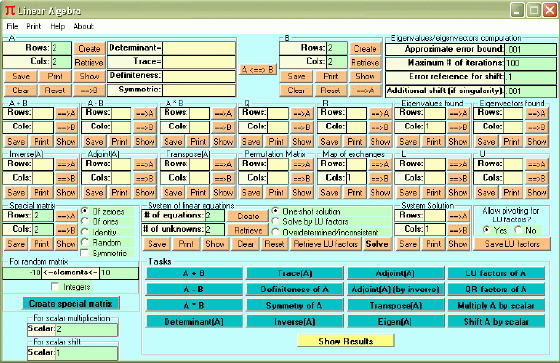## Linear Algebra

Linear Algebra performs computations associated with real matrices, including solution of linear systems of equations (even least squares solution of over-determined or inconsistent systems and solution by LU factors), matrix operations (add, ...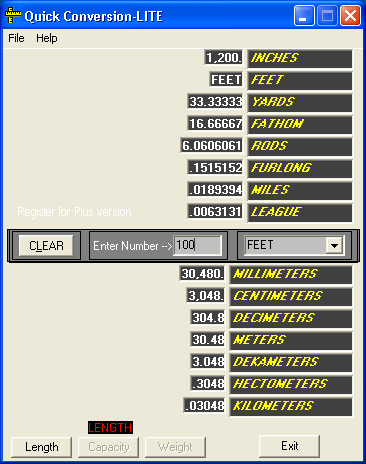## Quick Conversion

Quick Conversion is a piece of useful mathematics software that helps you convert units of length, weight and capacity conveniently. By typing a number into box provided will instantly display the results without the user having to search through a ...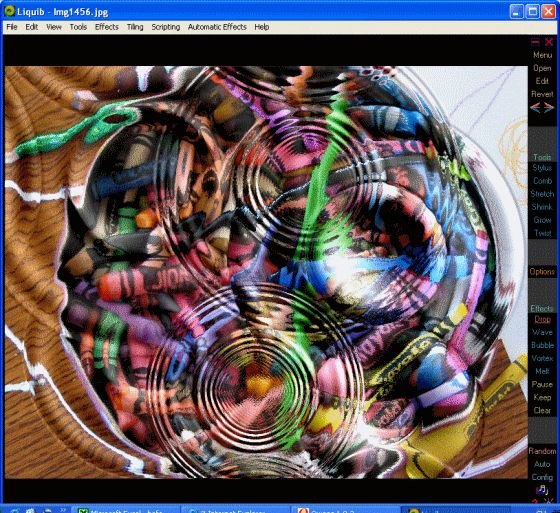## Liquib

Liquib treats an image as if it were a fluid, which can be distorted by various transformations to create liquid image and marbled paper type effects. It is a graphics tool (or toy) that attempts to translate mathematics into visual imagery.